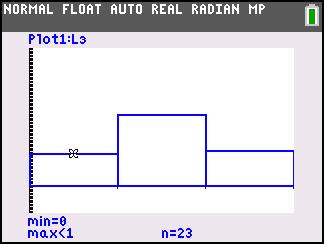### Independence is the Word

Students use a simulation to find the experimental probability of independent events.
•TI-84 Plus CE
•TI-84 Plus C Silver Edition
• TI-84 Plus Silver Edition
• TI-84 Plus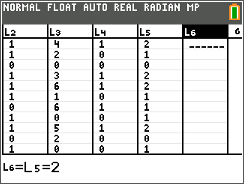### In It to Win It!

Students will calculate the experimental probability of winning a basketball game by shooting two free-throws using a random integer simulation.
•TI-84 Plus CE
•TI-84 Plus C Silver Edition
• TI-84 Plus Silver Edition
• TI-84 Plus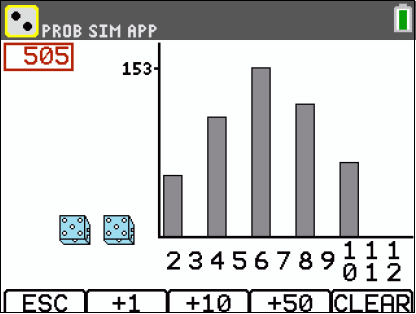### Roll the Dice

Students compute sample spaces for coin flips, find the various probabilities associated, and graph the probabilities using the calculator.
•TI-84 Plus CE
•TI-84 Plus C Silver Edition
• TI-84 Plus Silver Edition
• TI-84 Plus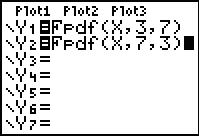### F Distribution

Students study the characteristics of the F distribution and discuss why the distribution is not symmetric (skewed right) and only has positive values.
•TI-84 Plus CE
•TI-84 Plus C Silver Edition
• TI-84 Plus Silver Edition
• TI-84 Plus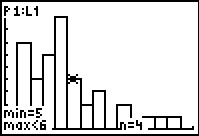### Geometric Distributions

Students simulate a geometric distribution of rolling a die to determine experimental probabilities and calculate theoretical probabilities.
•TI-84 Plus CE
•TI-84 Plus C Silver Edition
• TI-84 Plus Silver Edition
• TI-84 Plus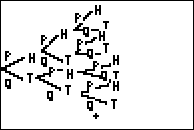### How Many? (Stats)

Students explore Bernoulli Probabilities. They will use them to calculate the probabilities of various single and cumulative events.
•TI-84 Plus CE
•TI-84 Plus C Silver Edition
• TI-84 Plus Silver Edition
• TI-84 Plus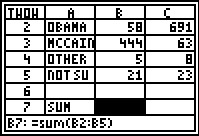### It's a Two-Way Street

Students will be introduced to two-way tables by calculating marginal and conditional distributions using formulas in a spreadsheet.
•TI-84 Plus CE
•TI-84 Plus C Silver Edition
• TI-84 Plus Silver Edition
• TI-84 Plus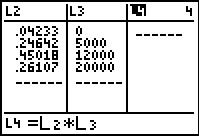### It's To Be Expected

Students use a tree diagram to find theoretical probabilities and use this information with lists to find the expected value.
•TI-84 Plus CE
•TI-84 Plus C Silver Edition
• TI-84 Plus Silver Edition
• TI-84 Plus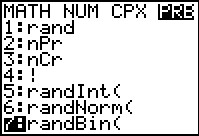### Makin' It Through The Winter

Students simulate a binomial distribution and calculate probabilities for a variety of situations involving binomial probability distributions.
•TI-84 Plus CE
•TI-84 Plus C Silver Edition
• TI-84 Plus Silver Edition
• TI-84 Plus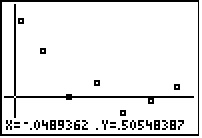### Probability Simulations

In this activity, students use the random integer (randInt) command to simulate probability experiments. They also graph the number of trials and corresponding probabilities to observe the Law of Large Numbers. Simulated experiments involve tossing a coin, spinning a spinner, and observing the sex of children in a family.
•TI-84 Plus CE
•TI-84 Plus C Silver Edition
• TI-84 Plus Silver Edition
• TI-84 Plus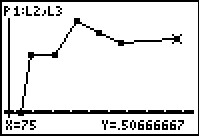### To Toss or To Simulate?

Students use the RandInt() command to simulate the tossing of a coin and the rolling of a die. They will collect data for several trials and use the information to investigate the Law of Large Numbers.
•TI-84 Plus CE
•TI-84 Plus C Silver Edition
• TI-84 Plus Silver Edition
• TI-84 Plus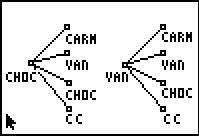••